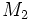# Minimal generating sets for a group may have different sizes

## Statement

It is possible to have a group$G$ (in fact, we can choose a finite cyclic group) with two subsets$M_1, M_2$ of$G$ such that:

•$M_1$ is a Minimal generating set (?) for$G$, i.e.,$M_1$ is a generating set for$G$ and removing any element from$M_1$ gives something that is not a generating set for$G$.
•$M_2$ is also a minimal generating set for$G$.
• The cardinalities of$M_1$ and$M_2$ are not equal.

## Related facts

A group where all minimal generating sets have the same size is termed a group in which all minimal generating sets have the same size. By Burnside's basis theorem, it turns out that any group of prime power order satisfies the property. There do exist groups that are not of prime power order which also satisfy the property; for instance, the symmetric group:S3 and more generally any dihedral group of odd prime degree has the property that every minimal generating set has size two.

## Proof

### Example of finite cyclic group of order six

Further information: cyclic group:Z6, element structure of cyclic group:Z6

Let$G := \mathbb{Z}/6\mathbb{Z}$ be the group of integers modulo 6 under addition. Consider$M_1 = \{ 1 \}$ and$M_2 = \{ 2,3 \}$. Both$M_1$ and$M_2$ are minimal generating sets and they have different sizes.